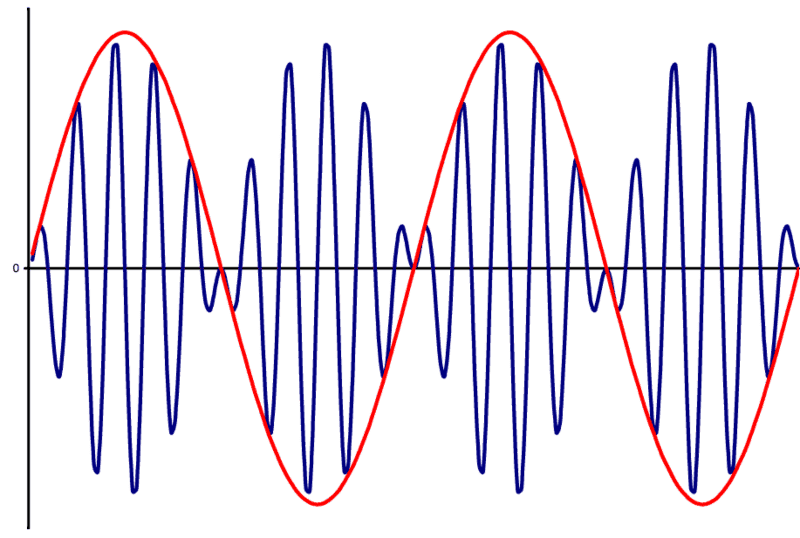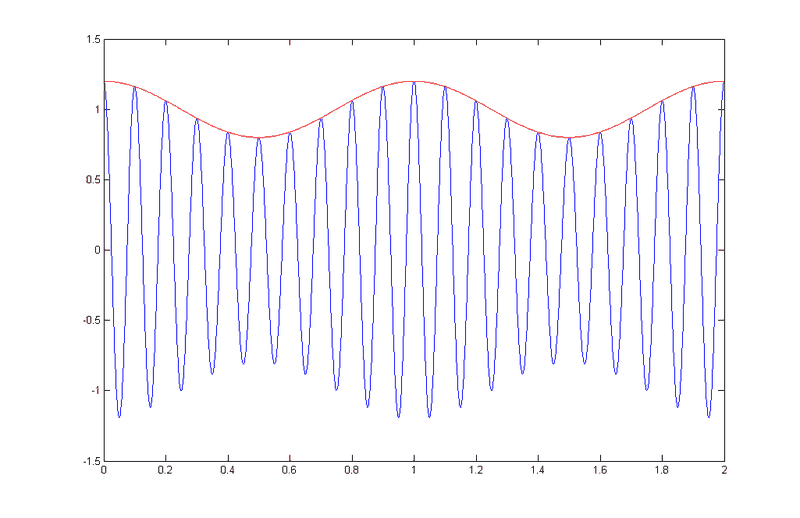# Amplitude modulation of signals with [suppressed] carrier

• Marvin94

#### Marvin94

Hello everybody, I'm a little bit confused about two types of amplitude modulation. We distinguish here:
Case I : Amplitude Modulation with suppressed carrier (https://en.wikipedia.org/wiki/Double-sideband_suppressed-carrier_transmission):Case II : Amplitude modulation with carrier:Now, it's not really clear to me the difference between the graphics above. More precisely, I have some questions about this topic:
1) How can be deduced from a signal plot which of the two cases is represented?
2) How can I understand the same from a matematical point of view? I mean, let's assume I have written down matematically the function of my signal. From this expression, how can I understand if the signal is modulated with carrier or with suppressed carrier?
3) Which operation should be done to obtain the Case I, and which operation to obtain the Case II ?

Thank you a lot in advance for any explanation!

It's often easier this consider these in the frequency domain. Assuming sinusoidal modulation, in the suppressed carrier case we simply have two pure tones (the sidebands) beating against each other. They could be, for example, 0.99MHz and 1.01MHz. Your Case I waveform could just as easily be representing two sound waves, close in frequency, beating against each other producing the wowwwow sound (say 499Hz and 501Hz).

In the second case there are three pure tones, the two sidebands, and the carrier which is centered in between the sidebands in frequency, (0.99MHz, 1MHz, 1.01MHz). You can see the carrier tone in the Case II waveform.

There are lots of references online describing the relevant math and the methods used to generate, for example:
https://en.wikipedia.org/wiki/Double-sideband_suppressed-carrier_transmission

In a simplified nutshell we can generate both of these by multiplying (with a mixer) the carrier tone (1MHz) with a modulation tone (10KHz) that is appropriately offset in amplitude. In the case of suppressed carrier the offset is zero [cos(ωmt)*cos(ωct)]. In the case of AM the offset is necessary [1+ cos(ωmt)]*cos(ωct)].

•Marvin94 and Averagesupernova
Good inputs to think about.. Thanks again !

In case 1, DSB, you will notice that the carrier phase reverses when the modulation crosses zero volts. That is because the DSB modulator is a “four quadrant” multiplier. Both carrier and modulation are bipolar signals that cross zero. Because the carrier flips phase by 180° it tends to cancel with itself over time, which suppresses the carrier, but the two sidebands remain.

In case 2, AM, the phase of the modulated carrier never reverses. The carrier is always present. The modulation signal is offset from zero sufficiently to always remains of the same polarity. The modulator used can then be a “two quadrant multiplier” and the receiver a very simple envelope detector.

•Marvin94 and the_emi_guy